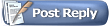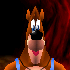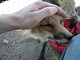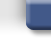Project64 Forumsretroben's n64 gameshark requests thread.
 Register FAQ Members List Calendar Search Today's Posts Mark Forums Read#1retrobenAlpha Tester Project Supporter Senior Member Join Date: Jul 2013 Posts: 686retroben's n64 gameshark requests thread.

Just request for any kind of code you can think of and I will try to make it happen.
#2the_randomizerAlpha Tester Project Supporter Senior Member Join Date: Sep 2008 Location: USA Posts: 1,136I just wish I actually understood hex, offsets, etc, then I'd actually be able to do cool hacks like you__________________
My rig:
CPU: Intel Core i7 4470 3.4 GHz to 3.9 GHz
Video card:: MSI nVidia GTX 970 4 GB GDDR5
OS: Windows 7 Professional 64-bit
RAM: 16 GB DDR3 SDRAM 10600
HDD: 2 x Western Digital 1 TB HDDs
Monitor: 23" Asus Full HD LED

Oh, and Snes9x > Zsnes in every way
#3retrobenAlpha Tester Project Supporter Senior Member Join Date: Jul 2013 Posts: 686Hexadecimals and you.

Elementary my dear Watson.

Hex numbers 0-F
0 1 2 3 4 5 6 7 8 9
A B C D E F
10 11 12...

Decimal numbers 0-9
0 1 2 3 4 5 6 7 8 9
10 11 12 13 14 15
16 17 18...

Additionally hex goes A0-FF as well.

Hex
A0=160 (16 x 10 = 160)

Decimal
160=160

Every tens place in hex equals sixteen.

Hex
10=16
16=22
1A=26
20=32 (decimals 16+16=32)

Decimal
10=10
16=16
20=20
22=22
26=26
32=32

Bits are in the same byte of an address.
8bits=1byte with ten placements.

The bits are as follows.

bit0? 0=0 neutral

bit1 1=1
bit2 2=2
bit3 4=4
bit4 8=8
bit5 10=16
bit6 20=32
bit7 40=64
bit8 80=128

bit9? FF=255 maximum (256th number including zero)

It can use any and every mixture of bits.
Example:bits 6,5,and 3 20+10+4=34 (52 in decimal).

00-7F in hex are the positive numbers.
80-FF in hex are the negative numbers.
Couning down from FF.
Examples:
FF= -1
FE= -2

80= -127 (maximum negative)
7F= +127 (maximum positive)

To think,I learned hex all on my own,not in school.

Last edited by retroben; 18th November 2013 at 06:04 AM.
#4the_randomizerAlpha Tester Project Supporter Senior Member Join Date: Sep 2008 Location: USA Posts: 1,136Quote:
 Originally Posted by retrobenElementary my dear Watson. Hex numbers 0-F 0 1 2 3 4 5 6 7 8 9 A B C D E F 10 11 12... Decimal numbers 0-9 0 1 2 3 4 5 6 7 8 9 10 11 12 13 14 15 16 17 18... Additionally hex goes A0-FF as well. Hex A0=160 (16 x 10 = 160) Decimal 160=160 Every tens place in hex equals sixteen. Hex 10=16 16=22 1A=26 20=32 (decimals 16+16=32) Decimal 10=10 16=16 20=20 22=22 26=26 32=32 Bits are in the same byte of an address. 8bits=1byte with ten placements. The bits are as follows. bit0? 0=0 neutral bit1 1=1 bit2 2=2 bit3 4=4 bit4 8=8 bit5 10=16 bit6 20=32 bit7 40=64 bit8 80=128 bit9? FF=255 maximum (256th number including zero) It can use any and every mixture of bits. Example:bits 6,5,and 3 20+10+4=34 (52 in decimal). To think,I learned hex all on my own,not in school.
I mean I know 0-255 in hex just fine, it's searching for the right values is the issue when looking at N64 games with the debugger. Then from there, figuring out what does what in the game and so on.
__________________
My rig:
CPU: Intel Core i7 4470 3.4 GHz to 3.9 GHz
Video card:: MSI nVidia GTX 970 4 GB GDDR5
OS: Windows 7 Professional 64-bit
RAM: 16 GB DDR3 SDRAM 10600
HDD: 2 x Western Digital 1 TB HDDs
Monitor: 23" Asus Full HD LED

Oh, and Snes9x > Zsnes in every way
#5retrobenAlpha Tester Project Supporter Senior Member Join Date: Jul 2013 Posts: 686What kind of codes would you be looking for?
#6the_randomizerAlpha Tester Project Supporter Senior Member Join Date: Sep 2008 Location: USA Posts: 1,136Quote:
 Originally Posted by retrobenWhat kind of codes would you be looking for?
Nothing in particular, just wanting to know how to look for them in general. I dunno....like the basics of searching values, reducing the number of results to manageable levels. Ugh, I don't know what I'm trying to sayJust navigating, getting the feeling of learning how to do this kind of hacking.
__________________
My rig:
CPU: Intel Core i7 4470 3.4 GHz to 3.9 GHz
Video card:: MSI nVidia GTX 970 4 GB GDDR5
OS: Windows 7 Professional 64-bit
RAM: 16 GB DDR3 SDRAM 10600
HDD: 2 x Western Digital 1 TB HDDs
Monitor: 23" Asus Full HD LED

Oh, and Snes9x > Zsnes in every way
#7HatCatAlpha Tester Project Supporter Senior Member Join Date: Feb 2007 Location: In my hat. Posts: 16,256You can search for decimal values in the memory debugger.
In fact, that's the default. You don't really need to know hexadecimal.

If you view the raw memory in RDRAM though, then you need to understand hex.
But, understanding base sixteen (i.e., hex), is pretty much the exact same as learning any other arithmetic base.

It's Third Grade knowledge, in fact. This stuff (not hex directly its self) was in the books when I was like, 8:
The decimal 666 is, in "extended notation", 6*(100) + 6*(10) + 6*(1).
More scientifically, 6*(10^2) + 6*(10^1) + 6*(10^0).
The base of each exponent is 10 because so-named "decimal" means ten digits.

If you already understand that then I think you pretty much already know hexadecimal. You just don't realize it.#8HatCatAlpha Tester Project Supporter Senior Member Join Date: Feb 2007 Location: In my hat. Posts: 16,256Quote:
 Originally Posted by retrobenBits are in the same byte of an address. 8bits=1byte with ten placements. The bits are as follows. bit0? 0=0 neutral bit1 1=1 bit2 2=2 bit3 4=4 bit4 8=8 bit5 10=16 bit6 20=32 bit7 40=64 bit8 80=128 bit9? FF=255 maximum (256th number including zero) It can use any and every mixture of bits. Example:bits 6,5,and 3 20+10+4=34 (52 in decimal).
Actually bits 6, 5, and 3 is 64+32+8, which is 104.
You said 52 which is only half of 104 because you got the bit offsets wrong.

First, there are only 8 bits in a byte, not 10.
There's no "bit8" and "bit9".
It's just bits 0 to 7, numbered little-endian.

Second, bit 0 is NOT "neutral".
2 to the power of 0 is 1, not 0.
So bit 0 means you mask | 1,
bit 1 means you mask | 2,
bit 2 means you mask | 4,
etc. all your values are off.Quote:
 Originally Posted by retroben00-7F in hex are the positive numbers. 80-FF in hex are the negative numbers. Couning down from FF. Examples: FF= -1 FE= -2 80= -127 (maximum negative) 7F= +127 (maximum positive)
This is true only if you assume two things:
• The corresponding integer storage is sign-extended (a "signed" int), to 8-bit precision.
• The CPU processor registering the numbers uses two's complement negation.

If it were a CPU besides MIPS/Intel it could be 1's complement negation, and negative numbers would not look exactly as you said.

More over, for our purposes, either in hacking or otherwise, a "byte" in a memory viewer should always default to an "unsigned char" perspective in C, so rather than sign-extending it, we're better off initially interpreting memory results as zero-extended bytes in the range 0:+255, not -128:+127.

Quote:
 Originally Posted by retrobenTo think,I learned hex all on my own,not in school.
Doesn't surprise me. It's at least what I did.

#9retrobenAlpha Tester Project Supporter Senior Member Join Date: Jul 2013 Posts: 686Zero is neutral because it is neither negative or positive.
There is no negative zero.

I know 8bits,ten positions make it decimal/hexadecimal.
I may have bit positions wrong,but my values are correct.

Every bit is multiples of two of the previous bit.

0
1 2 4 8 16 32 64 128
255

0
1 2 4 8 10 20 40 80
FF

I forgot to realize that 80 in hex can be positive when used
in half words (2 byte length).

A perfect example is the universal Sega Genesis
Sonic 1/2/3/K/3K games Ring Count Pro Action Replay code.

Rings=127
FFFE20:007F

Rings=128
FFFE20:0080

Rings=255
FFFE20:00FF

Rings=256
FFFE20:0100

Rings=999
FFFE20:03E7

The two byted amounts will stay positive until after 7FFF.

Rings=32767 (garbled counter)
FFFE20:7FFF

Values 80-FF in one byte codes can sometimes still be positive when used in item counts.

In physics based codes,80-FF are negative.
Running forward current speed is 32 (20 in hex).
Using the Zelda OOT Press A to Run Fast cheat modified as an example.
It might be two bytes long,which would need FFE0 for negative 32.
Change 20 to E0 and you will run backwards at 32 speed when trying to run forward.
Proof of zero being neutral,you will not move forward or backward with 0.
#10HatCatAlpha Tester Project Supporter Senior Member Join Date: Feb 2007 Location: In my hat. Posts: 16,256I don't care.
Bit 0 is not neutral.
It's a part of a byte, equally as much as every other bit.

Bits in a byte are numbered 0 to 7, not 1 to 10 or whatever.
So the correct answer was 104, not 52.

Quote:
 Originally Posted by retrobenI forgot to realize that 80 in hex can be positive when used in half words (2 byte length).
Halfwords are only 2 bytes on CPUs like MIPS.
A halfword can easily be 1 single byte on systems you haven't hacked for yet.
A halfword may also be 4 bytes or more for other systems.

To specify a negative hex, you use the negative sign, just as you would in decimal.

-0x80 is -128; +0x80 is +128.
Most often, a single byte in computer science is interpreted as "unsigned"; meaning it's 0:255, not -128:+127.

0xFFFF could be either +65535 or -1 if the 16-bit number is signed.
It depends which N64 CPU instructions buffer the number from RDRAM and how the programmer chooses to read it.

There is no universal rule of thumb that 0x8000:0xFFFF in 2 byte is ALWAYS a negative number, etc.Thread ToolsShow Printable VersionEmail this Page Display ModesLinear ModeSwitch to Hybrid ModeSwitch to Threaded ModePosting Rules You may not post new threads You may not post replies You may not post attachments You may not edit your posts BB code is On Smilies are On [IMG] code is On HTML code is Off
 Forum Jump User Control Panel Private Messages Subscriptions Who's Online Search Forums Forums Home General Discussion     Site News     Open Discussion Public Version     Project 64 - v2.x - Suggestions     Project 64 - v2.x - Issues     Project64 - Android     Project 64 - v1.6

All times are GMT. The time now is 11:32 AM.Contact Us - Archive - Top